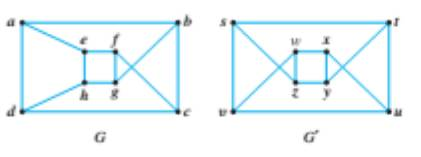Chapter 10.3, Problem 13ES### Discrete Mathematics With Applicat...

5th Edition
EPP + 1 other
ISBN: 9781337694193

#### Solutions

Chapter
Section### Discrete Mathematics With Applicat...

5th Edition
EPP + 1 other
ISBN: 9781337694193
Textbook Problem
1 views

# For each pair of graphs G and G’ in 6-13, determine whether G and G’ are isomorphic. If they are, give functions g: V(G) → V(G’) and h:E(G) → E(G’) that define the isomorphism. If they are not, give an invariant for graph isomorphism that they do not share.To determine

Whether G and G are isomorphic. If they are, give functions g:V(G)V(G) and h:E(G)E(G) that define the isomorphism. If they are not, give an invariant for graph isomorphism that they do not share.

Explanation

Given information:

Calculation:

Two graphs are G and G (with vertices V ( G ) and V(G) respectively and edges E ( G ) and E(G) respectively) are isomorphic if there exists one-to-one correspondence such that

[u,v] is an edge in G[g(u),g(v)] is an edge of G.

V(G) are all vertices of the graph GV(G')are all vertices of the graph G'

### Still sussing out bartleby?

Check out a sample textbook solution.

See a sample solution

#### The Solution to Your Study Problems

Bartleby provides explanations to thousands of textbook problems written by our experts, many with advanced degrees!

Get Started